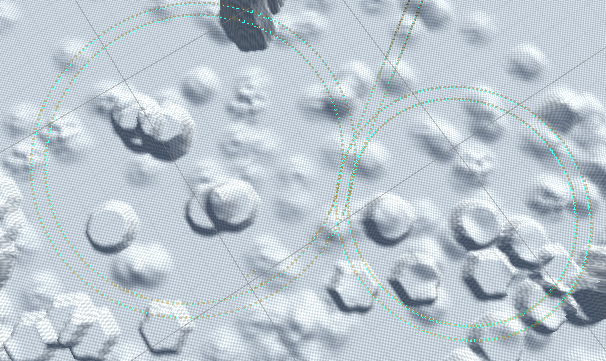# Rotation speeds are different despite having same code

When rotating my asset left or right (only holding one key down per direction) the circle the asset moves around in is bigger going left despite both directions having the same code. As you can see in the image below its not moving at the same rate when rotating left vs rotating right.Here is the code for it (you can ignore the vertical if else as they weren’t being used in the picture, and somehow work fine without issue compared to horizontals).

``````this.transform.position -= transform.forward * speed * boost* Time.deltaTime;

//this.transform.Rotate = Vector3.forward;
if(Input.GetAxisRaw("Vertical") > 0){
this.transform.Rotate(Vector3.right*1.5f*Time.deltaTime * rotationSpeed);
}else if(Input.GetAxisRaw("Vertical") < 0){
this.transform.Rotate(Vector3.left*1.5f*Time.deltaTime* rotationSpeed);
}
if(Input.GetAxisRaw("Horizontal") < 0){ //turn left (showing undesirable results)
this.transform.Rotate(Vector3.down*0.5f* rotationSpeed*Time.deltaTime);
//this.transform.Rotate(Vector3.back*1.8f*Time.deltaTime* rotationSpeed);
}else if(Input.GetAxisRaw("Horizontal") > 0){ //turn right (showing desired results)
this.transform.Rotate(Vector3.up*0.5f* rotationSpeed*Time.deltaTime);
//this.transform.Rotate(Vector3.forward*1.8f*Time.deltaTime* rotationSpeed);
}
``````

Solved: Position of the jet mesh was offset from the parent driving